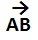# Scalars and Vectors

### Scalars and Vectors:

In the study of physics, we come across two types of physical quantities- Scalar and Vector. Scalar quantities, or simply scalars, are those which possess magnitude only whereas vector quantities, or simply vectors, are those which possess magnitude as well as direction.

For example- the distance between two places characterized by its magnitude only is a scalar quantity but the same characterized both by magnitude and direction is a vector quantity. To describe this more clearly, consider the distance between Bangalore and Delhi. It is a scalar quantity when we say that Bangalore is at a distance of 2000 km from Delhi. Now to locate the position of Bangalore, we should specify the direction in which the distance is to be measured and say that Bangalore is 2000 km south of Delhi. 2000 km south is a vector quantity. The distance and associated direction together determine a quantity called displacement. 2000 km south can, thus, be referred to as displacement. The following are some scalars and vectors:

Scalars: Distance, Time, Speed, Mass, Temperature, Pressure, Volume, Energy.

Vectors: Displacement, Velocity, Acceleration, Force, Momentum.

Vector is normally represented by drawing a line with an arrow. The length of the line, drawn according to some convenient scale, represents the magnitude of the vector while the arrow-head represents its direction. For illustration, consider a body moving with a velocity of 50 km/hour from north to south. We can represent the magnitude as well as the direction of this moving body by just drawing a line (AB) 5 cm long (5 cm = 50 km/hour, i.e. 1 cm = 10 km/hour) with an arrow pointing from north to south.

The displacement of the body from A to B can be denoted as-